NEET  >  33 Year NEET Previous Year Questions: Moving Charges & Magnetism - 1

# 33 Year NEET Previous Year Questions: Moving Charges & Magnetism - 1

Test Description

## 25 Questions MCQ Test Physics Class 12 | 33 Year NEET Previous Year Questions: Moving Charges & Magnetism - 1

33 Year NEET Previous Year Questions: Moving Charges & Magnetism - 1 for NEET 2023 is part of Physics Class 12 preparation. The 33 Year NEET Previous Year Questions: Moving Charges & Magnetism - 1 questions and answers have been prepared according to the NEET exam syllabus.The 33 Year NEET Previous Year Questions: Moving Charges & Magnetism - 1 MCQs are made for NEET 2023 Exam. Find important definitions, questions, notes, meanings, examples, exercises, MCQs and online tests for 33 Year NEET Previous Year Questions: Moving Charges & Magnetism - 1 below.
Solutions of 33 Year NEET Previous Year Questions: Moving Charges & Magnetism - 1 questions in English are available as part of our Physics Class 12 for NEET & 33 Year NEET Previous Year Questions: Moving Charges & Magnetism - 1 solutions in Hindi for Physics Class 12 course. Download more important topics, notes, lectures and mock test series for NEET Exam by signing up for free. Attempt 33 Year NEET Previous Year Questions: Moving Charges & Magnetism - 1 | 25 questions in 50 minutes | Mock test for NEET preparation | Free important questions MCQ to study Physics Class 12 for NEET Exam | Download free PDF with solutions
 1 Crore+ students have signed up on EduRev. Have you?
33 Year NEET Previous Year Questions: Moving Charges & Magnetism - 1 - Question 1

### A current carrying coil is subjected to a uniformmagnetic field. The coil will orient so that itsplane becomes 

Detailed Solution for 33 Year NEET Previous Year Questions: Moving Charges & Magnetism - 1 - Question 1

The plane of coil will orient itself so that area vector aligns itself along the magnetic field.
So, the plane will orient perpendicular to the magnetic field.

33 Year NEET Previous Year Questions: Moving Charges & Magnetism - 1 - Question 2

### Tesla is the unit of               

Detailed Solution for 33 Year NEET Previous Year Questions: Moving Charges & Magnetism - 1 - Question 2

Tesla is the unit of magnetic field.

33 Year NEET Previous Year Questions: Moving Charges & Magnetism - 1 - Question 3

### Energy in a current carrying coil is stored in theform of             

Detailed Solution for 33 Year NEET Previous Year Questions: Moving Charges & Magnetism - 1 - Question 3

Energy is stored in magnetic field.

33 Year NEET Previous Year Questions: Moving Charges & Magnetism - 1 - Question 4

The total charge induced in a conducting loopwhen it is moved in a magnetic field depends on         

Detailed Solution for 33 Year NEET Previous Year Questions: Moving Charges & Magnetism - 1 - Question 4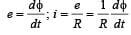Total charge induced =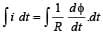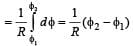33 Year NEET Previous Year Questions: Moving Charges & Magnetism - 1 - Question 5

The magnetic induction at a point P which is at adistance of 4 cm from a long current carryingwire is 10–3 T. The field of induction at a distance12 cm from the current will be 

Detailed Solution for 33 Year NEET Previous Year Questions: Moving Charges & Magnetism - 1 - Question 5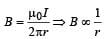As the distance is increased to three times, the magnetic induction reduces to one third.
Hence,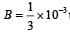tesla = 3.33 x 10-4 tesla

33 Year NEET Previous Year Questions: Moving Charges & Magnetism - 1 - Question 6

A deuteron of kinetic energy 50 keV is describinga circular orbit of radius 0.5 metre in a planeperpendicular to the magnetic field B. The kineticenergy of the proton that describes a circularorbit of radius 0.5 metre in the same plane withthe same B is        

Detailed Solution for 33 Year NEET Previous Year Questions: Moving Charges & Magnetism - 1 - Question 6

For a charged particle orbiting in a circular path in a magnetic field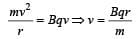or, mv2 = Bqvr

Also,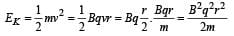For deuteron,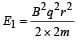For proton,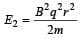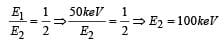33 Year NEET Previous Year Questions: Moving Charges & Magnetism - 1 - Question 7

A uniform magnetic field acts at right angles tothe direction of motion of electron. As a result,the electron moves in a circular path of radius2cm. If the speed of electron is doubled, thenthe radius of the circular path will be 

Detailed Solution for 33 Year NEET Previous Year Questions: Moving Charges & Magnetism - 1 - Question 7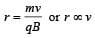As v is doubled, the radius also becomes double. Hence, radius = 2 × 2 = 4 cm

33 Year NEET Previous Year Questions: Moving Charges & Magnetism - 1 - Question 8

The magnetic field at a distance r from a longwire carrying current i is 0.4 tesla. The magneticfield at a distance 2r is                

Detailed Solution for 33 Year NEET Previous Year Questions: Moving Charges & Magnetism - 1 - Question 8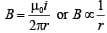When r is doubled, the magnetic field becomes half, i.e., now the magnetic field will be 0.2 T.

33 Year NEET Previous Year Questions: Moving Charges & Magnetism - 1 - Question 9

A straight wire of length 0.5 m that is carrying a current of 1.2 A is placed in a uniform magnetic field of induction 2T, and the magnetic field is perpendicular to the length of the wire. What is the force of the wire?

Detailed Solution for 33 Year NEET Previous Year Questions: Moving Charges & Magnetism - 1 - Question 9

F = Biℓ = 2 ×1.2 × 0.5 = 1.2 N

33 Year NEET Previous Year Questions: Moving Charges & Magnetism - 1 - Question 10

To convert a galvanometer into an ammeter, oneneeds to connect a        

Detailed Solution for 33 Year NEET Previous Year Questions: Moving Charges & Magnetism - 1 - Question 10

To convert a galvanometer into an ammeter, one needs to connect a low resistance in parallel so that maximum current passes through the shunt wire and ammeter remains protected

33 Year NEET Previous Year Questions: Moving Charges & Magnetism - 1 - Question 11

A coil carrying electric current is placed inuniform magnetic field, then         

Detailed Solution for 33 Year NEET Previous Year Questions: Moving Charges & Magnetism - 1 - Question 11

A current carrying coil has magnetic dipole moment. Hence, a torque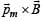acts on it in magnetic field.

33 Year NEET Previous Year Questions: Moving Charges & Magnetism - 1 - Question 12

A charge moving with velocity v in X-directionis subjected to a field of magnetic induction innegative X-direction. As a result, the charge will              

Detailed Solution for 33 Year NEET Previous Year Questions: Moving Charges & Magnetism - 1 - Question 12

The force acting on a charged particle in magnetic field is given by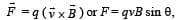When angle between v and B is 180°, F = 0

33 Year NEET Previous Year Questions: Moving Charges & Magnetism - 1 - Question 13

An electron enters a region where magnetic field(B) and electric field (E) are mutually perpendicular,then        

Detailed Solution for 33 Year NEET Previous Year Questions: Moving Charges & Magnetism - 1 - Question 13

When the deflection produced by electric field is equal to the deflection produced by magnetic field, then the electron can go undeflected.

33 Year NEET Previous Year Questions: Moving Charges & Magnetism - 1 - Question 14

A straight wire of diameter 0.5 mm carrying acurrent of 1 A is replaced by another wire of 1mm diameter carrying same current. The strengthof magnetic field far away is          [1995, 97, 99]

Detailed Solution for 33 Year NEET Previous Year Questions: Moving Charges & Magnetism - 1 - Question 14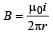and so it is independent of thickness.
The current is same in both the wires, hence magnetic field induced will be same.

33 Year NEET Previous Year Questions: Moving Charges & Magnetism - 1 - Question 15

At what distance from a long straight wirecarrying a current of 12 A will the magnetic fieldbe equal to 3×10-6Wb /m2 ?        

Detailed Solution for 33 Year NEET Previous Year Questions: Moving Charges & Magnetism - 1 - Question 15

Current (I) = 12 A and magnetic field (B) = 3 × 10–5 Wb/m2. Consider magnetic field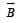at distance r..

Magnetic field ,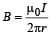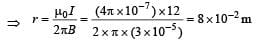33 Year NEET Previous Year Questions: Moving Charges & Magnetism - 1 - Question 16

The magnetic field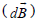due to a small element (dℓ ) at a distance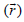and element carrying current i is           

Detailed Solution for 33 Year NEET Previous Year Questions: Moving Charges & Magnetism - 1 - Question 16

According to Biot Savart law,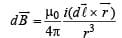33 Year NEET Previous Year Questions: Moving Charges & Magnetism - 1 - Question 17

A 10 eV electron is circulating in a plane at rightangles to a uniform field at magnetic induction10– 4 Wb/m2 (= 1.0 gauss). The orbital radius ofthe electron is 

Detailed Solution for 33 Year NEET Previous Year Questions: Moving Charges & Magnetism - 1 - Question 17

K.E. of electron = 10 eV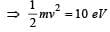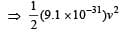= 10 x 1.6 x 10 -19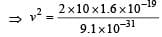⇒ v2 = 3.52 × 1012 ⇒ v = 1.88 × 106 m

Also, we know that for circular motion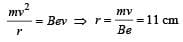33 Year NEET Previous Year Questions: Moving Charges & Magnetism - 1 - Question 18

Two equal electric currents are flowing perpendicular to each other as shown in the figure. AB and CD are perpendicular to each other and symmetrically placed with respect to the current flow. Where do we expect the resultant magnetic field to be zero?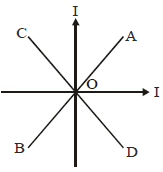Detailed Solution for 33 Year NEET Previous Year Questions: Moving Charges & Magnetism - 1 - Question 18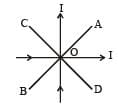Net magnetic field on AB is zero because magnetic field due to both current carrying wires is equal in magnitude but opposite in direction.

33 Year NEET Previous Year Questions: Moving Charges & Magnetism - 1 - Question 19

A beam of electrons is moving with constantvelocity in a region having simultaneousperpendicular electric and magnetic fields ofstrength 20 Vm–1 and 0.5 T respectively at rightangles to the direction of motion of the electrons.Then the velocity of electrons must be         

Detailed Solution for 33 Year NEET Previous Year Questions: Moving Charges & Magnetism - 1 - Question 19

The electron moves with constant velocity without deflection. Hence, force due to magnetic field is equal and opposite to force due to electric field.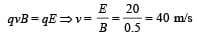33 Year NEET Previous Year Questions: Moving Charges & Magnetism - 1 - Question 20

A galvanometer of resistance 20 Ω gives fullscale deflection with a current of 0.004 A. Toconvert it into an ammeter of range 1 A, therequired shunt resistance should be          

Detailed Solution for 33 Year NEET Previous Year Questions: Moving Charges & Magnetism - 1 - Question 20

Maximum current which can pass through galvanometer,  Ig = 0.004A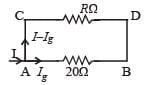Let R be the resistance of shunt.
We know potential drop across AB = Potential drop across CD R (I – Ig) = Ig (20)
⇒ R (1 – 0.004) = 0.004 × 20 ⇒ R = 0.08Ω

33 Year NEET Previous Year Questions: Moving Charges & Magnetism - 1 - Question 21

A positively charged particle moving due eastenters a region of uniform magnetic field directedvertically upwards. The particle will      

Detailed Solution for 33 Year NEET Previous Year Questions: Moving Charges & Magnetism - 1 - Question 21

In a perpendicular magnetic field, the path of a charged particle is a circle, and the magnetic field does not cause any change in energy.

33 Year NEET Previous Year Questions: Moving Charges & Magnetism - 1 - Question 22

Two long parallel wires are at a distance of 1metre. Both of them carry one ampere of current.The force of attraction per unit length betweenthe two wires is 

Detailed Solution for 33 Year NEET Previous Year Questions: Moving Charges & Magnetism - 1 - Question 22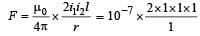= 2 × 10–7 N/m. [This relates to the definition of ampere]

33 Year NEET Previous Year Questions: Moving Charges & Magnetism - 1 - Question 23

A galvanometer having a resistance of 8 ohmsis shunted by a wire of resistance 2 ohms. If thetotal current is 1 amp, the part of it passingthrough the shunt will be          

Detailed Solution for 33 Year NEET Previous Year Questions: Moving Charges & Magnetism - 1 - Question 23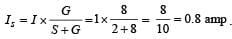33 Year NEET Previous Year Questions: Moving Charges & Magnetism - 1 - Question 24

A coil of one turn is made of a wire of certainlength and then from the same length a coil oftwo turns is made. If the same current is passedin both the cases, then the ratio of the magneticinductions at their centres will be 

Detailed Solution for 33 Year NEET Previous Year Questions: Moving Charges & Magnetism - 1 - Question 24

Let ℓ be length of wire.
Ist case : ℓ =2πr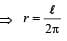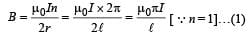2nd Case : ℓ = 2(2πr')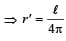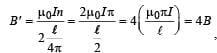using (1) (where n = 2)

33 Year NEET Previous Year Questions: Moving Charges & Magnetism - 1 - Question 25

Magnetic field intensity at the centre of a coil of50 turns, radius 0.5 m and carrying a current of 2A is       

Detailed Solution for 33 Year NEET Previous Year Questions: Moving Charges & Magnetism - 1 - Question 25

We know that magnetic field at the centre of circular coil,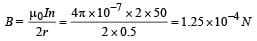## Physics Class 12

157 videos|452 docs|213 tests
 Use Code STAYHOME200 and get INR 200 additional OFF Use Coupon Code
Information about 33 Year NEET Previous Year Questions: Moving Charges & Magnetism - 1 Page
In this test you can find the Exam questions for 33 Year NEET Previous Year Questions: Moving Charges & Magnetism - 1 solved & explained in the simplest way possible. Besides giving Questions and answers for 33 Year NEET Previous Year Questions: Moving Charges & Magnetism - 1, EduRev gives you an ample number of Online tests for practice

## Physics Class 12

157 videos|452 docs|213 tests

### How to Prepare for NEET

Read our guide to prepare for NEET which is created by Toppers & the best Teachers